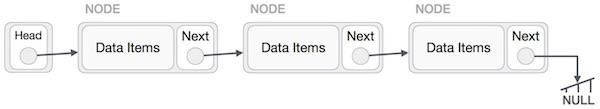# Sum of the alternate nodes of linked list in C++

In this problem, we are given a linked list. Our task is to print the sum of alternate nodes of the linked list.

Linked list is a sequence of data structure which are connected together via links.Now, let’s get back to the problem. Here, we will add alternate nodes of the linked list. This means we will add nodes are positions 0, 2, 4, 6, …

Let’s take an example to understand the problem,

Input

4 → 12 → 10 → 76 → 9 → 26 → 1

Output

24

Explanation

considering alternate strings −
4 + 10 + 9 + 1 = 24

To solve this problem, we will visit each node one by one and for every nest node. We will add value to the sum. To keep a check on nodes, we will use a flag.

This can be done using iteration or recursion. We will discuss both here,

## Example

Iterative approach

Live Demo

#include <iostream>
using namespace std;
struct Node {
int data;
struct Node* next;
};
void pushNode(struct Node** head_ref, int newData) {
struct Node* newNode = (struct Node*)malloc(sizeof(struct Node));
newNode->data = newData;
}
bool flag = true;
int sum = 0;
if (flag)
flag = !flag;
}
return sum;
}
int main(){
cout<<"The sum of alternate nodes is "<<sumAlternateNodeIt(head);
return 0;
}

## Output

The sum of alternate nodes is 24

## Example

Recursive Approach

Live Demo

#include <iostream>
using namespace std;
struct Node {
int data;
struct Node* next;
};
void pushNode(struct Node** head_ref, int new_data){
struct Node* new_node = (struct Node*)malloc(sizeof(struct Node));
new_node->data = new_data;
}
void sumAlternateNodeRec(struct Node* node, int& sum, bool flag = true){
if (node == NULL)
return;
if (flag == true)
sum += (node->data);
sumAlternateNodeRec(node->next, sum, !flag);
}
int main(){
}
The sum of alternate nodes is 24